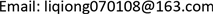1湖北理工学院，湖北 黄石

2华中科技大学，水电与数字化工程学院，湖北 武汉1. 引言

2. 传统灰色聚类模型

x i ( s ) = ∑ e = 1 15 x i e ( s ) (1)

r i e ( s ) = x i e ( s ) x i ( s ) (2)

r i s = ( r i 1 ( s ) , r i 2 ( s ) , ⋯ , r i 15 ( s ) ) (3)

R ( s ) = ( r 1 , 1 ( s ) r 1 , 2 ( s ) ⋯ r 1 , 5 ( s ) r 2 , 1 ( s ) r 2 , 2 ( s ) ⋯ r 2 , 5 ( s ) ⋮ ⋮ ⋱ ⋮ r 15 , 1 ( s ) r 15 , 2 ( s ) ⋯ r 15 , 5 ( s ) ) (4)

B ( s ) = U R ⋅ R ( s ) = ( b 1 ( s ) , b 2 ( s ) , ⋯ , b 5 ( s ) ) (5)

3. 模型改进3.1. 指数型白化函数

3.2. 基于熵的聚类权确定

u i j = d i j ∑ i = 1 m d i j (6)

e j = − k ∑ i = 1 m u i j ln u i j (7)

w j = ( 1 − e j ) ( m − ∑ j = 1 n e j ) (8)

σ i k = ∑ j = 1 n f j k ( d i j ) w j (9)

4. 应用实例4.1. 山洪灾害风险区划评价对象及数据

Hazard assessment data for mountain torrent

Vulnerability assessment data for mountain torrent

4.2. 用改进灰色聚类法确定评价对象灰类及风险等级

4.2.1. 建立指数形白化权函数进行综合评价确定评价对象灰类

Continued

f 1 = { 1 x ∈ [ 0 , 10 ] e 10 − x 10 x ∈ ( 10 , + ∞ ) f 2 = { e x − 10 10 x ∈ [ 0 , 10 ] 1 x ∈ ( 10 , 70 ] e 70 − x 70 x ∈ ( 70 , + ∞ ) f 3 = { e x − 70 70 x ∈ [ 0 , 70 ] 1 x ∈ ( 70 , 100 ] e 100 − x 100 x ∈ ( 100 , + ∞ )

f 4 = { e x − 100 100 x ∈ [ 0 , 100 ] 1 x ∈ ( 100 , 150 ] e 150 − x 150 x ∈ ( 150 , + ∞ ) f 5 = { 1 x ∈ ( 150 , + ∞ ) x − 125 125 x ∈ [ 0 , 150 ]

4.2.2. 计算灰色评价权矩阵

x i ( s ) = ∑ e = 1 15 x i e ( s ) (6)

r i e ( s ) = x i e ( s ) x i ( s ) (7)

r i s = ( r i 1 ( s ) , r i 2 ( s ) , ⋯ , r i 15 ( s ) ) (8)

R ( s ) = ( r 1 , 1 ( s ) r 1 , 2 ( s ) ⋯ r 1 , 5 ( s ) r 2 , 1 ( s ) r 2 , 2 ( s ) ⋯ r 2 , 5 ( s ) ⋮ ⋮ ⋱ ⋮ r 15 , 1 ( s ) r 15 , 2 ( s ) ⋯ r 15 , 5 ( s ) ) (9)

5. GIS软件的应用

6. 山洪灾害风险分析

R = 0.8 H + 0.2 V

Risk classification of mountain torrents in Hubei Provinc

Risk evaluation result

7. 结论与展望